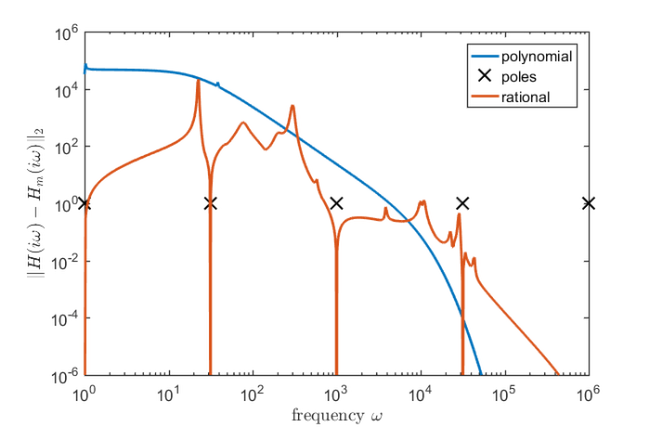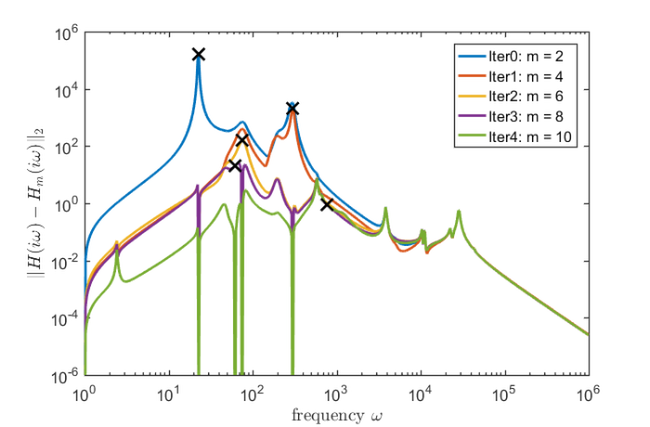# CD player model order reduction

## Introduction

Consider a linear time invariant multi-input multi-output system described by the state space equations

$\displaystyle \dot{x}(t) = A x(t) + B u(t),$

and

$\displaystyle y(t) = C x(t),$

where $x(t)$ denotes the state vector of length $n$ and $u(t), y(t)$ denote the input and output vectors of length $p$. The large sparse matrix $A$ is of size $n\times n$ and $B, C^T$ are of size $n \times p$.

Block rational Krylov spaces  are a powerful tool for model order reduction provided a good set of poles is chosen. Here we explore different choices of poles, and compare how well the the reduced model behaves compared to the original system.

## CD player

We start by loading the CD player problem, and constructing a function handle for the transfer function of the system:

$\displaystyle H(s) = C(s I_{n} - A)^{-1} B.$

For each set of poles, we construct the approximate transfer function

$\displaystyle H_m(s) = C\mathbf{V}_m (s I_{ms} - \mathbf{V}_m^* A \mathbf{V}_m)^{-1} \mathbf{V}_m^* B.$

We compare the two models by plotting the error

$\displaystyle \| H(s) - H_m(s) \|_2$

for $s$ over the range of $i [10^0, 10^6]$.

if exist('CDplayer.mat') ~= 2
disp(['The required matrix for this problem can be downloaded from '  ...
'http://slicot.org/20-site/126-benchmark-examples-for-model-reduction']);
return
end
H = @(s) C*((s*speye(size(A)) - A)\B);


## Infinite poles vs equally spaced poles

We compare a block polynomial Krylov approximation against a block rational Krylov approximation. The polynomial space is constructed with 10 poles set to infinity, whereas the rational space has 5 poles logarithmically spaced in the interval $i[10^0, 10^6]$, complemented with their complex conjugates to obtain a real block rational Arnoldi decomposition. For both approaches, we plot the norm of the difference between the transfer function and the approximate tranfer function.

% block polynomial Krylov space
xi = inf*ones(1,10);
V = rat_krylov(A,B,xi);

Am = V'*A*V;
Cm = C*V;
Bm = V'*B;
Hm = @(s) Cm*((s*speye(size(Am)) - Am)\Bm);
Xm = @(s) V*((s*eye(size(Am)) - Am)\(V'*B));
Gam = @(s) B - (s*speye(size(A)) - A)*Xm(s);

errHm = [];
s = 1i*logspace(0,6,500);
for k = 1:length(s)
errHm(k) = norm(H(1i*s(k)) - Hm(1i*s(k)));
end

loglog(imag(s), errHm, 'LineWidth',2); hold on
xlabel('frequency $\omega$', 'Interpreter', 'latex')
ylabel('$\|H(i \omega) - H_m(i \omega)\|_2$', 'Interpreter', 'latex')

% block rational Krylov space
xi = 1i*logspace(0,6,5);
xi = util_cplxpair(xi, conj(xi));
param.real = 1;
V = rat_krylov(A,B,xi);

Am = V'*A*V;
Cm = C*V;
Bm = V'*B;
Hm = @(s) Cm*((s*speye(size(Am)) - Am)\Bm);

errHm = [];
s = union(xi, 1i*logspace(0,6,500));
for k = 1:length(s)
errHm(k) = norm(H(s(k)) - Hm(s(k)));
end

p = loglog(imag(xi), ones(1, length(xi)), 'kx', 'MarkerSize', 12);
loglog(imag(s), errHm, 'LineWidth',2); axis([0, 1e6, 1e-6, 1e6])
xlabel('frequency $\omega$', 'Interpreter', 'latex')
ylabel('$\|H(i \omega) - H_m(i \omega)\|_2$', 'Interpreter', 'latex')
legend({'polynomial', 'poles', 'rational'})In this example we start with two poles at $[1i, -1i]$. We then select the following poles adaptively, in complex conjugate pairs, using the procedure described in Section 3.2 in . This procedure proves to be quite effective, with the error curve dropping significantly from iteration to iteration. Note that we are using the extension functionality of the rat_krylov function, which allows to extend an existing block rational Arnoldi decomposition with new block basis vectors.

cand = 1i*logspace(0,6,500);
xi = [ 1i , conj(1i) ];
param.real = 1;
[V, K_, H_] = rat_krylov(A, B, xi, param); % initial run

param.extend = 2;  % allow for the iterative extension of BRAD
figure()
for iter = 1:5
Am = V'*A*V;
Bm = V'*B;
Cm = C*V;
Hm = @(s) Cm*((s*speye(size(Am)) - Am)\Bm);
Xm = @(s) V*((s*eye(size(Am)) - Am)\(V'*B));
Gam = @(s) B - (s*speye(size(A)) - A)*Xm(s);
errHm = [];
for k = 1:length(cand)
nrmGam(k) = norm(Gam(cand(k)));
errHm(k) = norm(H(cand(k)) - Hm(cand(k)));
end
mem(iter)=loglog(imag(cand),errHm, 'LineWidth',2);
hold on, shg

[val,ind] = max(nrmGam);
loglog(imag(cand(ind)), errHm(ind), 'kx', 'MarkerSize', 12, 'LineWidth', 2)
xi = [ cand(ind) , conj(cand(ind)) ];
[V,K_,H_] = rat_krylov(A, V, K_, H_, xi, param); % extend decomposition
end

legend(mem,{'Iter0: m = 2', 'Iter1: m = 4', 'Iter2: m = 6', ...
'Iter3: m = 8', 'Iter4: m = 10'})
xlabel('frequency $\omega$', 'Interpreter', 'latex')
ylabel('$\|H(i \omega) - H_m(i \omega)\|_2$', 'Interpreter', 'latex')
axis([0, 1e6, 1e-6, 1e6])## References

 O. Abidi, M. Hached, and K. Jbilou. Adaptive rational block Arnoldi methods for model reductions in large-scale MIMO dynamical systems, New Trends Math. Sci., 4(2):227--239, 2016

 S. Elsworth and S. Güttel. The block rational Arnoldi method, SIAM J. Matrix Anal. Appl., 41(2):365--388, 2020.